Related Articles
Functions in MATLAB
• Difficulty Level : Hard
• Last Updated : 24 Feb, 2021

Methods are also popularly known as functions. The main aim of the methods is to reuse the code. A method is a block of code which is invoked and executed when it is called by the user. It contains local workspace and independent of base workspace which belongs to command prompt. Let’s take a glance of method syntax.

Syntax:

function [y1, y2 ,y3 . . . . , yn] = functionName(arguments)

. . . . .

end

where, y1 . . . . yn are output variables.

MATLAB syntax is quite peculiar compared to other programming languages. We can return one or more values from a function. We can also pass one or more arguments/variables while calling a function. MATLAB functions must be defined in separate files and function name must match with the file name. Let’s also see the few more ways of defining a function as per the user needs.

• Anonymous Functions
• Sub Functions
• Nested Functions
• Private Functions

Now let’s dive into an example and understand how to define a basic function.

Example:

## MATLAB

 `% A MATLAB program to illustrate``% defining a funtcion`` ` `function` `result = adder(x, y, z)`` ` `% This function adds the 3 input arguments``result = x+y+z;``end`

The comment line that is written just after the function statement works as the help text. Save the above code as adder.m and observe the output by calling it from the command prompt.

Output: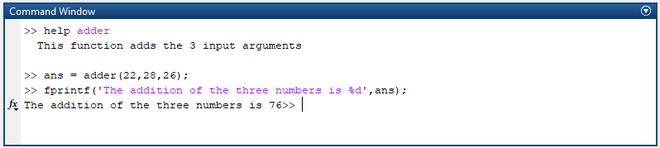Calling the user defined function

## Anonymous Functions

An Anonymous function is as an inline function with one output variable. It can contain multiple input and output arguments. A user can’t access/call an anonymous function from outside the file. User can define an anonymous function in the command prompt or within a script or function file.

Syntax:

output = @(arguments) expression

Parameters:

output  = output to be returned

arguments = required inputs to be passed

expression = a single formula/logic to be

Example: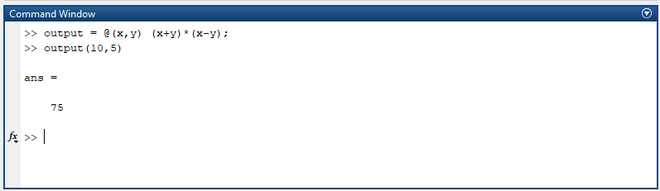Output

In the above block of code, an anonymous function is defined and accessed in the command prompt itself.

## Sub Functions

Sub functions are the functions that are defined after a primary function. Every function must be defined in a file except anonymous functions. Sub functions are defined in the primary function file and these functions are not visible to any other functions except the primary and sub functions that are defined within the file. Unlike primary functions, sub-functions can’t be accessed from command prompt/another file.

Syntax:

function output = mainFunction(x)

. . . . . .

subFunction(y)

. . . . . .

end

function result = subFunction(y)

. . . . . .

end

Example:

## MATLAB

 `% Printing the sum of two numbers``% using sub functions`` ` `% Primary Function``function` `result = adder(x,y)``result = x+y;`` ` `% Calling Sub function``print(result);``end`` ` `% Sub function``function` `print(result)``fprintf(``'The addition of given two number is %d'``,result);``end`

Save the above code with the primary function name adder.m and observe the output by calling it from the command prompt.

Output: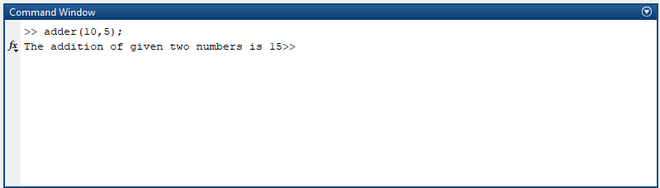Adding Two Numbers

## Nested Functions

Unlike Sub functions, Nested functions are defined inside the primary functions. The scope of a nested function is within the file. One can’t access the nested function from outside the file. Primary function’s workspace can be accessed by all the nested functions that are defined within the body of the primary function.

## MATLAB

 `% A MATLAB program to illustrate nested functions`` ` `% Primary Function``function` `result = adder(x,y)``result = x+y;`` ` `% Nested Function``function` `print(result)``fprintf(``'The sum of two numbers added in the nested function %d'``,result);``end`` ` `% Calling Nested Function``print(result);``end`

Save the above code with the primary file name adder.m and observe the output by calling the function.

Output: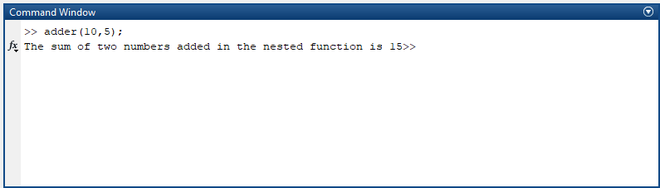Result

## Private Functions

The name itself suggests that these functions are private and will only be visible to limited functions/files. These functions files are stored in a separate subfolder named private. Generally, we can’t access files that are not in the current path/folder. But this works for private functions. These functions reside in subfolder and accessible to the parent folder and private folder itself. Now let’s dive into some example to understand the concept better.

Example:

## MATLAB

 `% A MATLAB program to illustrate``% private functions`` ` `% Adder Function``function` `result = adder(x,y)``result = x+y;`` ` `% Calling private function``print(result);``end`

Save the above code as adder.m in separate folder and create one more folder called private.

## MATLAB

 `% A MATLAB program to illustrate``% private functions`` ` `% private function``function` `print(result)``fprintf(``'The sum of two numbers added in the nested function is %d'``,result);``end`

Save the above code as print.m and save it in the private folder.

## MATLAB

 `% A MATLAB program to illustrate``% private functions`` ` `% Subtractor Function``function` `result = adder(x,y)``result = x-y;`` ` `% Calling private function``print(result);``end`

Save the above code as subtractor.m and store it in outside of parent folder. Now let’s observe the output by accessing the above functions.

Output: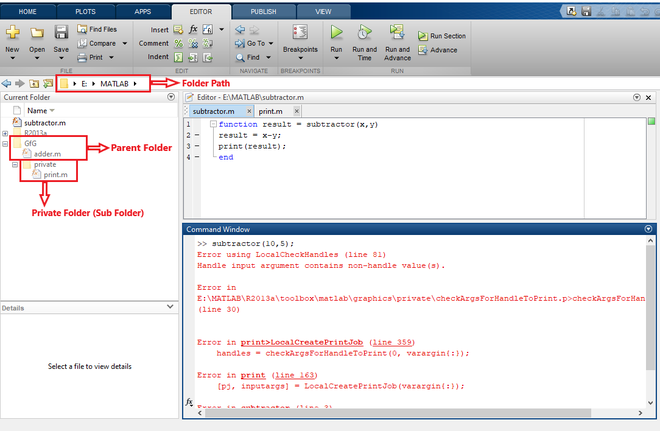Calling private function from subtractor function

In the above case, you can observe the error when we try to access private functions from the location other than the parent folder.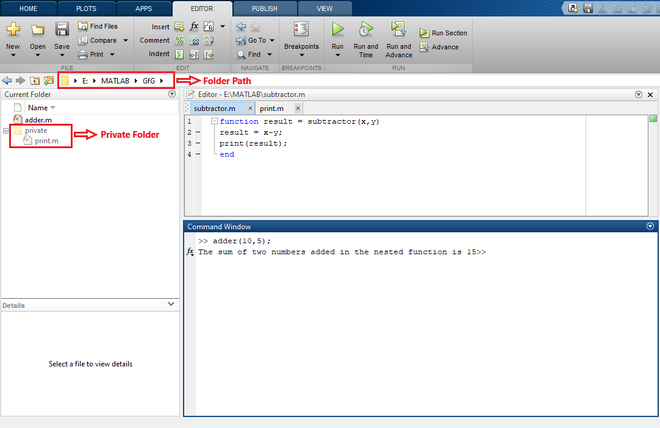Calling private function from adder function

The program compiled successfully and gave the output when we tried to access the private function from adder function which is stored in the parent folder. One must check the current file path while working on private functions.

Attention reader! Don’t stop learning now. Get hold of all the important CS Theory concepts for SDE interviews with the CS Theory Course at a student-friendly price and become industry ready.

My Personal Notes arrow_drop_up